1. 电子科技大学 机械与电气工程学院, 成都 611731;
2. 清华大学 机械工程系, 北京 100084;
3. 武昌理工学院 信息工程学院, 武汉 430223

Rolling-type micro-vibration isolation mechanisms based on elastic contact
GUAN Gaofeng1, XU Dengfeng2, ZHU Yu1,2, YU Qiang1, LI Qiang3
1. School of Mechanical and Electrical Engineering, University of Electronic Science and Technology of China, Chengdu 611731, China;
2. Department of Mechanical Engineering, Tsinghua University, Beijing 100084, China;
3. School of Engineering Information, Wuchang University of Technology, Wuhan 430223, China
Abstract: Modeling of rolling-type mechanisms for micro-vibration isolation is usually based on the assumption of rigid contact and pure rolling motion that leads to large errors in the measured and theoretical natural frequencies. This study presents a model using elastic contact with elastic deformation and micro-sliding between the contact surfaces that leads to a differential equation for the system motion based on Lagrange's equation to predict the natural frequency. Previous models have only contained structural parameters while this one also includes related material parameters. The analysis predicts natural frequencies that are larger than those in previous models because the elastic potential energy stored in the contact interfaces increases the total potential energy. The theoretical results agree much better with measurements with errors of less than 25% compared to previous models that had errors of 70%.
Key words: micro-vibration isolation     rolling-type mechanism     natural frequency     elastic contact

Harvey等于2016年对近些年的研究进行了综述，从建模方式来看，前述文献中对于不同结构形式的滚动隔振机构的研究均是基于刚性接触和纯滚动的假设，然后通过Newton第二定律或Lagrarge方程得到系统运动微分方程，进而得到固有频率表达式。在上述假设下进行建模，模型仅仅牵涉到机构的结构参数。从实验或仿真结果来看，固有频率指标是上述研究中关注的焦点，但是在地面微幅振动隔离实验中，该指标普遍存在较大偏差。文中，理论固有频率0.46 Hz，实测固有频率1 Hz，偏差大于100%。文中，理论固有频率0.62 Hz，实测固有频率约为1.25 Hz，偏差大于100%。文所提机构理论固有频率1 Hz，实测固有频率约为3.5 Hz，偏差约为250%。

1 基于刚性接触的滚动隔振机构模型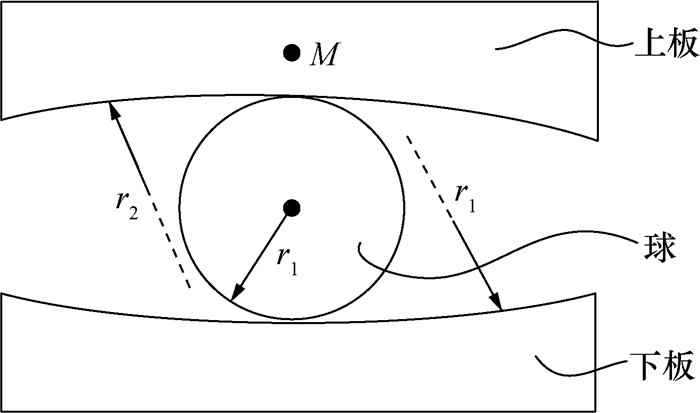图 1 典型的滚动隔振机构

 $2\left( {{r_2} - {r_1}} \right)\ddot \alpha + g\alpha = 0.$ (1)

 $f = \frac{1}{{2{\rm{ \mathsf{ π} }}}}\sqrt {\frac{g}{{2\left( {{r_2} - {r_1}} \right)}}} .$ (2)

2 基于弹性接触的滚动隔振机构建模 2.1 球与球接触时的弹性蠕滑率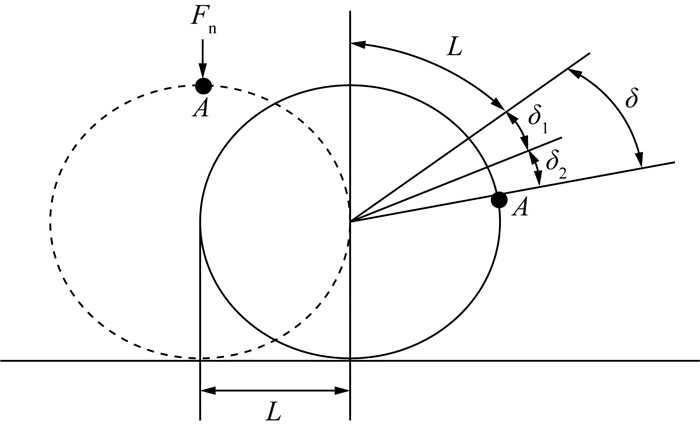图 2 弹性变形和微观滑动示意图

 $\xi = \frac{\delta }{L} = \frac{{\mu T}}{{ - 2{r_1}{F_{\rm{n}}}}}.$ (3)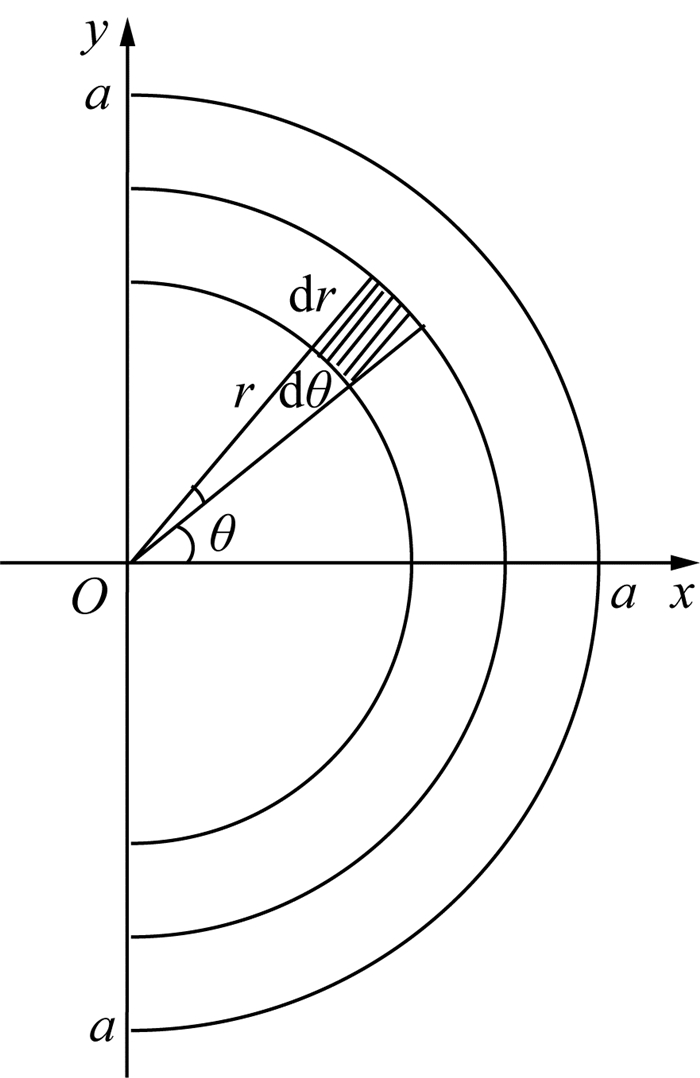图 3 滚动阻力矩的求解示意图

 $T = \int_{ - {\rm{ \mathsf{ π} }}/2}^{{\rm{ \mathsf{ π} }}/2} {\int_{ - a}^a {{r^2}\cos \theta p\left( r \right){\rm{d}}r{\rm{d}}\theta } } = {\rm{ \mathsf{ π} }}{p_0}{a^3}/8.$ (4)

 $p\left( r \right) = \frac{{{p_0}}}{a}{\left( {{a^2} - {r^2}} \right)^{1/2}}.$ (5)

 $a = {\left( {\frac{{3{F_{\rm{n}}}r}}{{4E}}} \right)^{1/3}},$ (6)
 ${p_0} = {\left( {\frac{{6{F_{\rm{n}}}{E^2}}}{{{{\rm{ \mathsf{ π} }}^3}{r^2}}}} \right)^{1/3}}.$ (7)

 $E = \frac{{{E_1}{E_2}}}{{{E_1} + {E_2}}},$ (8)
 $r = \frac{{{r_1}{r_2}}}{{{r_2} - {r_1}}}.$ (9)

 $\xi = \frac{\delta }{L} = \frac{\mu }{{{r_1}}} \times \frac{3}{{32}}{\left( {\frac{{6r{F_{\rm{n}}}}}{E}} \right)^{1/3}}.$ (10)
2.2 滚动隔振机构的动力学建模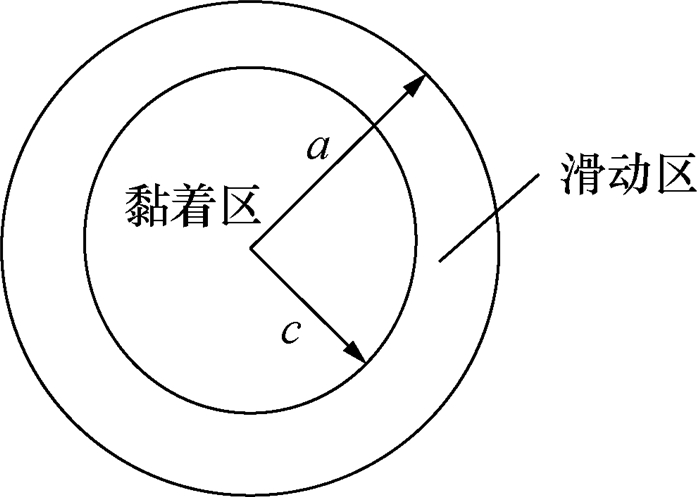图 4 接触面中黏着区与滑动区的划分

 $\frac{c}{a} = {\left( {\frac{{\mu {F_{\rm{n}}} - {F_{\rm{x}}}}}{{\mu {F_{\rm{n}}}}}} \right)^{1/3}}.$ (11)

 $\delta = {\delta _1} + {\delta _2}.$ (12)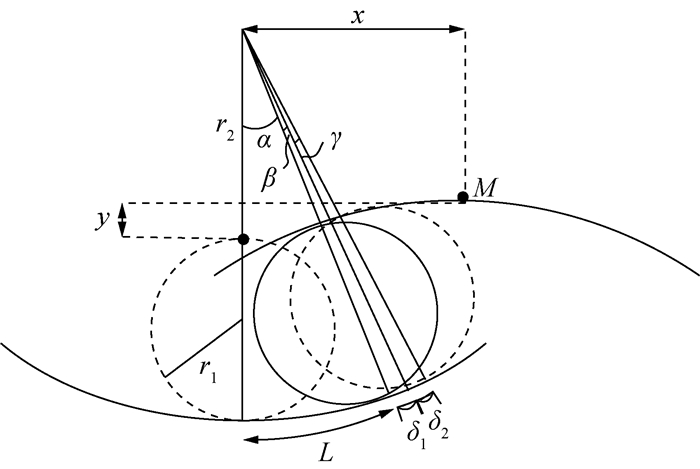图 5 非平衡位置时机构的几何关系

 $\alpha :\beta :\gamma = L:{\delta _1}:{\delta _2}.$ (13)

 $x = 2\left( {{r_2} - {r_1}} \right)\sin \alpha ,$ (14)
 $y = 2\left( {{r_2} - {r_1}} \right)\left( {1 - \cos \alpha } \right).$ (15)

 $T = \frac{1}{2}M\left( {{{\dot x}^2} + {{\dot y}^2}} \right).$ (16)

 $\mathit{V} = {\mathit{V}_1} + {\mathit{V}_2}.$ (17)

 ${\mathit{V}_1} = Mgy.$ (18)

 ${\mathit{V}_2} = 2 \times \frac{1}{2}{k_{\rm{t}}}\delta _1^2.$ (19)

 ${k_{\rm{t}}} = \frac{{8Ga}}{{\left( {2 - v} \right)}}.$ (20)

 $G = \frac{E}{{2\left( {1 + v} \right)}}.$ (21)

 $\frac{{\rm{d}}}{{{\rm{d}}t}}\left( {\frac{{\partial \left( {T + V} \right)}}{{\partial \alpha }}} \right) - \frac{{\partial \left( {T + V} \right)}}{{\partial \alpha }} = 0.$ (22)

 $M\left( {{r_2} - {r_1}} \right)\ddot \alpha + \left[ {Mg + \left( {{r_2} - {r_1}} \right){k_{\rm{t}}}{{\left( {{\delta _1}/L} \right)}^2}} \right]\alpha = 0.$ (23)

 $f = \frac{1}{{2{\rm{ \mathsf{ π} }}}}\sqrt {\frac{{Mg + \left( {{r_2} - {r_1}} \right){k_1}{{\left( {{\delta _1}/L} \right)}^2}}}{{2M\left( {{r_2} - {r_1}} \right)}}} .$ (24)
2.3 微幅振动情况下滚动隔振机构的固有频率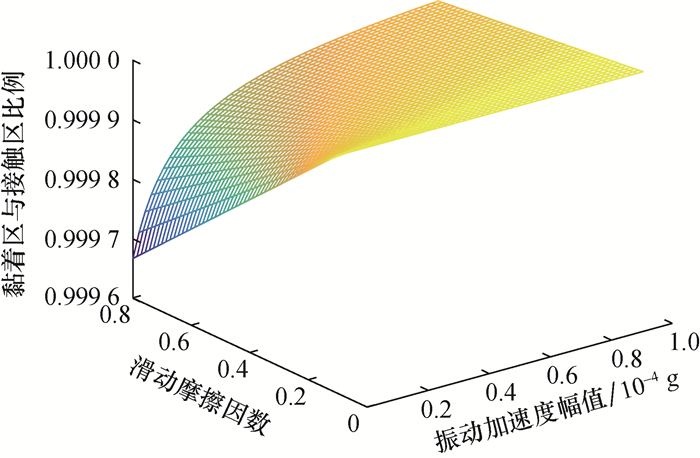图 6 (网络版彩图)黏着区与接触区之比分析

 $\delta \approx {\delta _1}.$ (25)

 $\xi \approx \frac{{{\delta _1}}}{L}.$ (26)

 $f = \frac{1}{{2{\rm{ \mathsf{ π} }}}}\sqrt {\frac{g}{{2\left( {{r_2} - {r_1}} \right)}} + \frac{{27g{r_2}{\mu ^2}}}{{512{r_1}\left( {{r_2} - {r_1}} \right)\left( {2 - v} \right)\left( {1 + v} \right)}}} .$ (27)

3 实验验证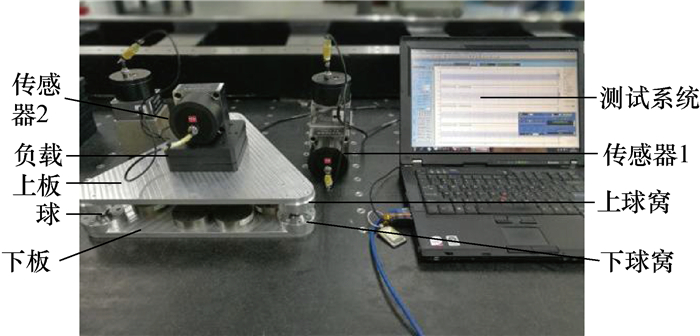图 7 实验装置图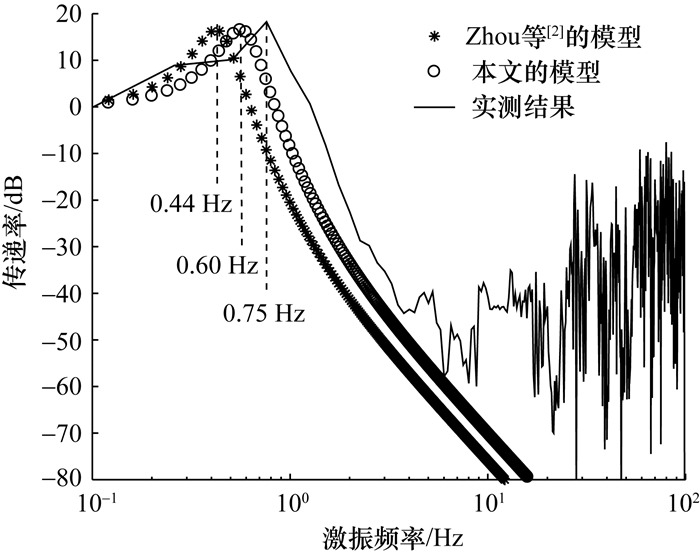图 8 理论结果与实测结果的对比

4 结论

  LIN T W, HONE C C. Base isolation by free rolling rods under basement[J]. Earthquake Engineering & Structural Dynamics, 1993, 22(3): 261-273.  ZHOU Q, LU X L, WANG Q M, et al. Dynamic analysis on structures base-isolated by a ball system with restoring property[J]. Earthquake Engineering & Structural Dynamics, 1998, 27(8): 773-791.  JANGID R S, LONDHE Y B. Effectiveness of elliptical rolling rods for base isolation[J]. Journal of Structural Engineering, 1998, 124(4): 469-472. DOI:10.1061/(ASCE)0733-9445(1998)124:4(469)  JANGID R S. Stochastic seismic response of structures isolated by rolling rods[J]. Engineering Structures, 2000, 22(8): 937-946. DOI:10.1016/S0141-0296(99)00041-3  YASUDA M, MINBU S, PAN G, et al. Vibration control unit and vibration control body: US, 7278623B2. 2007-10-09.  KEMENY Z A. Micro vibration isolation device: USA, 6517060B1. 2003-02-11.  BUTTERWORTH J W. Seismic response of a non-concentric rolling isolator system[J]. Advances in Structural Engineering, 2006, 9(1): 39-54. DOI:10.1260/136943306776232918  CHUNG L L, YANG C Y, CHEN H M, et al. Dynamic behavior of nonlinear rolling isolation system[J]. Structural Control and Health Monitoring, 2009, 16(1): 32-54. DOI:10.1002/stc.305  GANG G F, YU Q, SHEN F. Theory and experiments of a rolling-ball mechanism for horizontal vibration isolation[C]//Proceedings of 2015 Joint International Mechanical, Electronic and Information Technology Conference. Chongqing, China: Atlantis Press, 2015: 846-852.  HARVEY JR P S, KELLY K C. A review of rolling-type seismic isolation:Historical development and future directions[J]. Engineering Structures, 2016, 125: 521-531. DOI:10.1016/j.engstruct.2016.07.031  波波夫.接触力学与摩擦学的原理及其应用[M].李强, 雒建斌, 译.北京: 清华大学出版社, 2011. POPOV V L. Contact mechanics and friction physical principles and applications[M]. LI Q, LUO J B, trans. Beijing: Tsinghua University Press, 2011. (in Chinese)  金学松.轮轨蠕滑理论及其试验研究[D].成都: 西南交通大学, 1999. JIN X S. Study on creep theory of wheel and rail system and its experiment[D]. Chengdu: Southwest Jiaotong University, 1999. (in Chinese)  张俊杰, 马勇. 双盘式渐开线仪中的轮轨弹性蠕滑问题[J]. 机械传动, 2007, 31(5): 6-8. ZHANG J J, MA Y. Elastical creepages between wheel and rail in double-disk involute measuring apparatus[J]. Journal of Mechanical Transmission, 2007, 31(5): 6-8. DOI:10.3969/j.issn.1004-2539.2007.05.002 (in Chinese)  HARVEY JR P S, GAVIN H P. The nonholonomic and chaotic nature of a rolling isolation system[J]. Journal of Sound and Vibration, 2013, 332(14): 3535-3551. DOI:10.1016/j.jsv.2013.01.036  SUBRAHMANYAN P K. A model approach to precision motion control[D]. Cambridge, Massachusetts, USA: Massachusetts Institute of Technology, 1999.